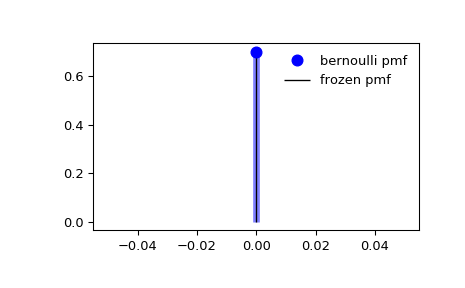# scipy.stats.bernoulli¶

scipy.stats.bernoulli(*args, **kwds) = <scipy.stats._discrete_distns.bernoulli_gen object>[source]

A Bernoulli discrete random variable.

As an instance of the rv_discrete class, bernoulli object inherits from it a collection of generic methods (see below for the full list), and completes them with details specific for this particular distribution.

Notes

The probability mass function for bernoulli is:

$\begin{split}f(k) = \begin{cases}1-p &\text{if } k = 0\\ p &\text{if } k = 1\end{cases}\end{split}$

for $$k$$ in $$\{0, 1\}$$.

bernoulli takes $$p$$ as shape parameter.

The probability mass function above is defined in the “standardized” form. To shift distribution use the loc parameter. Specifically, bernoulli.pmf(k, p, loc) is identically equivalent to bernoulli.pmf(k - loc, p).

Examples

>>> from scipy.stats import bernoulli
>>> import matplotlib.pyplot as plt
>>> fig, ax = plt.subplots(1, 1)


Calculate a few first moments:

>>> p = 0.3
>>> mean, var, skew, kurt = bernoulli.stats(p, moments='mvsk')


Display the probability mass function (pmf):

>>> x = np.arange(bernoulli.ppf(0.01, p),
...               bernoulli.ppf(0.99, p))
>>> ax.plot(x, bernoulli.pmf(x, p), 'bo', ms=8, label='bernoulli pmf')
>>> ax.vlines(x, 0, bernoulli.pmf(x, p), colors='b', lw=5, alpha=0.5)


Alternatively, the distribution object can be called (as a function) to fix the shape and location. This returns a “frozen” RV object holding the given parameters fixed.

Freeze the distribution and display the frozen pmf:

>>> rv = bernoulli(p)
>>> ax.vlines(x, 0, rv.pmf(x), colors='k', linestyles='-', lw=1,
...         label='frozen pmf')
>>> ax.legend(loc='best', frameon=False)
>>> plt.show()Check accuracy of cdf and ppf:

>>> prob = bernoulli.cdf(x, p)
>>> np.allclose(x, bernoulli.ppf(prob, p))
True


Generate random numbers:

>>> r = bernoulli.rvs(p, size=1000)


Methods

 rvs(p, loc=0, size=1, random_state=None) Random variates. pmf(k, p, loc=0) Probability mass function. logpmf(k, p, loc=0) Log of the probability mass function. cdf(k, p, loc=0) Cumulative distribution function. logcdf(k, p, loc=0) Log of the cumulative distribution function. sf(k, p, loc=0) Survival function (also defined as 1 - cdf, but sf is sometimes more accurate). logsf(k, p, loc=0) Log of the survival function. ppf(q, p, loc=0) Percent point function (inverse of cdf — percentiles). isf(q, p, loc=0) Inverse survival function (inverse of sf). stats(p, loc=0, moments=’mv’) Mean(‘m’), variance(‘v’), skew(‘s’), and/or kurtosis(‘k’). entropy(p, loc=0) (Differential) entropy of the RV. expect(func, args=(p,), loc=0, lb=None, ub=None, conditional=False) Expected value of a function (of one argument) with respect to the distribution. median(p, loc=0) Median of the distribution. mean(p, loc=0) Mean of the distribution. var(p, loc=0) Variance of the distribution. std(p, loc=0) Standard deviation of the distribution. interval(alpha, p, loc=0) Endpoints of the range that contains alpha percent of the distribution

#### Previous topic

scipy.stats.random_correlation

#### Next topic

scipy.stats.betabinom